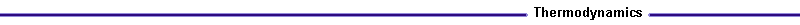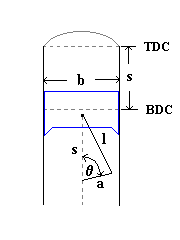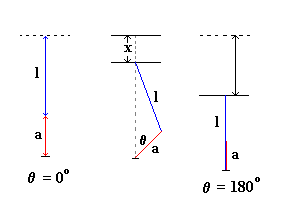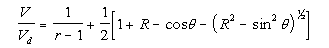Slider Crank ModelLink to: Piston Cylinder Volume Applet

Derivation of Slider-Crank Model

The volume of the piston cylinder can be determined as a function of crank angle from the compression ratio, the stroke, bore and connecting rod length. The geometric parameters of the piston cylinder are represented in Figure 1.Figure 1. Piston Cylinder
Where:
b = bore
s = stroke
l = connecting rod length
a = crank radius (= ½ s)
theta = crank angle
TDC = top dead center
BDC = bottom dead center

Top dead center refers to the position of the crank shaft at an crank angle of 0o. This position is otherwise known as the clearance volume, Vc. At bottom dead center the crank angle is at 180 o. In this position the cylinder volume is at its maximum, V1. The difference between the maximum and minimum volume, V1- Vo, is defined as the displacement volume, Vd. The displacement volume can also be represented as a function of the bore and stroke:(1)
At a given crank angle the volume is given by :
V = Vc + p /4 b2 (2)Figure 2. Piston Cylinder Geometry
Again using geometry, a relationship for x can be developed:Figure 3. Geometric solution for x(3)
The compression ratio is defined as the ratio between the maximum and minimum volume, r = V1/Vo. For Otto ignition  r = 10 and for a diesel engine the compression ration varies from 12 to 24. Substituting the maximum volume with the displacement volume yields:
r = 1 + Vd/Vc (4)
Solving for Vc results in:
Vo = Vd / r-1 (5)
Substituting equations (3) and (5) into equation (2), results in the following relationship for the cylinder volume.(6)
Where:
R = l/a

The final form of the Slider-Crank model is given as a non-dimensional relationship by dividing both sides of equation (6) by Vd.(7)
Click here to see how the volume varies with the crank angle: Volume Applet

Derivation of Surface Area of the Piston Cylinder

In order to study the effect of heat transfer within the piston cylinder, the surface area of the cylinder has to be evaluated. The combustion chamber surface area is given by:(8)
Where:
Ach = cylinder head surface area
Ap = piston head area

The equation can be further approximated by:(9)